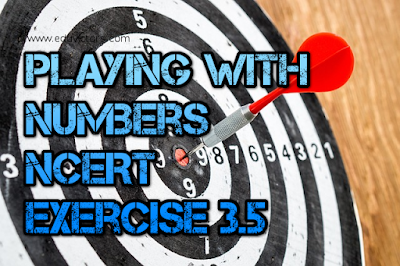## Saturday, 29 April 2017

### CBSE Class 6 Maths - Playing With Numbers - NCERT Exercise 3.5 (#cbsenotes)

Playing With Numbers

## EXERCISE 3.5

CBSE Class 6 Maths

Q1: Which of the following statements are true?
(a) If a number is divisible by 3, it must be divisible by 9.
(b) If a number is divisible by 9, it must be divisible by 3.
(c) A number is divisible by 18, if it is divisible by both 3 and 6.
(d) If a number is divisible by 9 and 10 both, then it must be divisible by 90.
(e) If two numbers are co-primes, at least one of them must be prime.
(f) All numbers which are divisible by 4 must also be divisible by 8.
(g) All numbers which are divisible by 8 must also be divisible by 4.
(h) If a number exactly divides two numbers separately, it must exactly divide their sum.
(i) If a number exactly divides the sum of two numbers, it must exactly divide the two numbers separately.

(a) (e.g. 15 is divisible by 3 but not by 9)
(b)
(c)
(d)
(e) (e.g. 8 and 9 are co-primes and none of these are prime. )
(f)
(g)
(h)
(i)

Q2: Here are two different factor trees for 60. Write the missing numbers.

Q3: Which factors are not included in the prime factorisation of a composite number?

Answer: 1 is not included in the prime factorisation of a composite number. (∵ 1 is neither prime nor composite)

Q4: Write the greatest 4-digit number and express it in terms of its prime factors.

Answer: The greatest 4-digit number = 9999

9999
↙ ↘
3 3333
↙ ↘
3  1111
↙ ↘
11 101
∴ The prime factors of 9999 = 3 × 3 × 11 × 101

Q5: Write the smallest 5-digit number and express it in the form of its prime factors.

Answer: The smallest five digit number is 10000.

10000
↙ ↘
2  5000
↙ ↘
2  2500
↙ ↘
5  500
↙ ↘
5  100
↙ ↘
5  20
↙ ↘
5  4
↙ ↘
2  2

The prime factors of 10000 are 2 × 2 × 2 × 2 × 5 × 5 × 5 × 5.

Q6: Find all the prime factors of 1729 and arrange them in ascending order. Now state the relation, if any; between two consecutive prime factors.

Answer: Prime factors of 1729 = 7 × 13 × 19
The difference of any two consecutive prime numbers = 6
i.e. 13 - 7 = 6, 19 - 13 = 6

Q7: The product of three consecutive numbers is always divisible by 6. Verify this statement with the help of some examples.

Answer: Among the three consecutive numbers, at least one number is even and one is multiple of 3. Thus, the product must be multiple of 6.

e.g.
2 × 3 × 4 = 24
6 × 7 × 8 = 336

Q8: The sum of two consecutive odd numbers is divisible by 4. Verify this statement with the help of some examples.

3 + 5 = 8 and 8 is divisible by 4.
5 + 7 = 12 and 12 is divisible by 4.
7 + 9 = 16 and 16 is divisible by 4.
9 + 11 = 20 and 20 is divisible by 4

Q9: In which of the following expressions, prime factorisation has been done?

(a) 24 = 2 × 3 × 4
(b) 56 = 7 × 2 × 2 × 2
(c) 70 = 2 × 5 × 7
(d) 54 = 2 × 3 × 9

(a) ∵ 4 is not a prime factor.
(b)
(c)
(d) ∵ 9 is not a prime factor.

Q10: Determine if 25110 is divisible by 45.
[Hint : 5 and 9 are co-prime numbers. Test the divisibility of the number by 5 and 9].

Prime factors of 45 = 5 × 9
25110 is divisible by 5 as ‘0’ is at its unit place.
25110 is divisible by 9 as sum of digits (2+5+1+1+0 = 9) is divisible by 9.
∴ the number must be divisible by 5 × 9 = 45

Q11: 18 is divisible by both 2 and 3. It is also divisible by 2 × 3 = 6. Similarly, a number is divisible by both 4 and 6. Can we say that the number must also be divisible by 4 × 6 = 24? If not, give an example to justify your answer.

Answer: No. Number 12 is divisible by both 6 and 4 but 12 is not divisible by 24.

Q12: I am the smallest number, having four different prime factors. Can you find me?

Answer: The smallest four prime numbers are 2, 3, 5 and 7.
Hence, the required number is 2 × 3 × 5 × 7 = 210